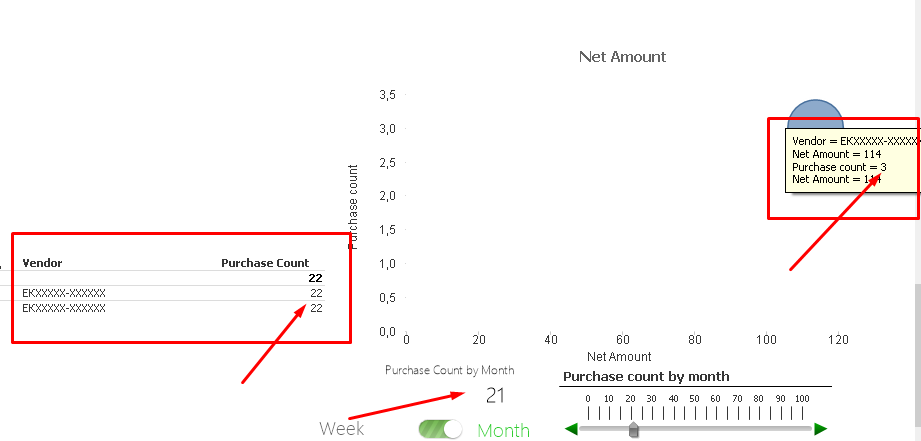New to QlikView

Discussion board where members can get started with QlikView.

Announcements
New Contributor III

Problem with scatter chart

Hello guys,

I have problems with my scatter chart. Here it is:There is an obvious difference in the amounts.

Here are my formulas:

DIMENSIONS

- Vendor

=if(vNSMonth>0 and vNSWeek=0,

(if(aggr(Count(DISTINCT Invoice_V),Year,Month,Vendor_Name)>=vNSMonth,Vendor_Name)),

if(vNSMonth=0 and vNSWeek>0,

(if(aggr(Count(DISTINCT Invoice_V),Year,Month,Week,Vendor_Name)>=vNSWeek,Vendor_Name))

))

EXPRESSIONS

- Net Amount(X axis): Sum ([NetAmount_V])

- Purchase count(Y axis😞 count (Distinct [Invoice_V])

- Net Amount(Z axis😞 Sum ([NetAmount_V])

Thanks,

Todor

Tags (2)